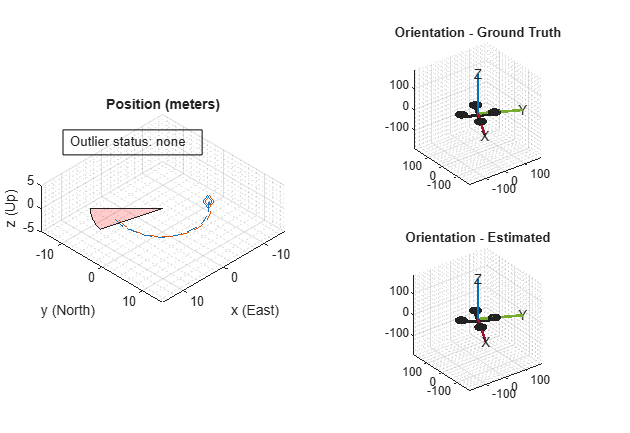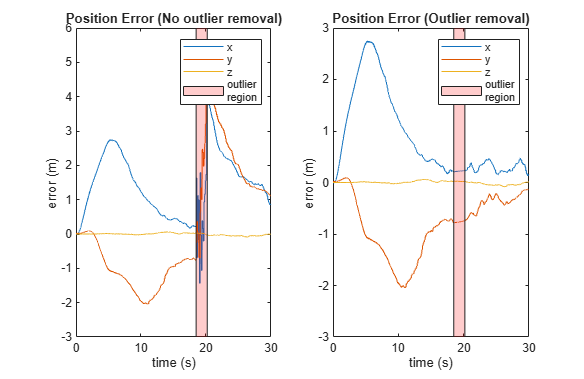# Detect Noise in Sensor Readings with Residual Filtering

This example shows how to use the `residualgps` object function and residual filtering to detect when new sensor measurements may not be consistent with the current filter state.

### Load Trajectory and Sensor Data

Load the MAT-file `loggedDataWithMultipath.mat`. This file contains simulated IMU and GPS data as well as the ground truth position and orientation of a circular trajectory. The GPS data contains errors due to multipath errors in one section of the trajectory. These errors were modelled by adding white noise to the GPS data to simulate the effects of an urban canyon.

```load('loggedDataWithMultipath.mat', ... 'imuFs', 'accel', 'gyro', ... % IMU readings 'gpsFs', 'lla', 'gpsVel', ... % GPS readings 'truePosition', 'trueOrientation', ... % Ground truth pose 'localOrigin', 'initialState', 'multipathAngles') % Number of IMU samples per GPS sample. imuSamplesPerGPS = (imuFs/gpsFs); % First and last indices corresponding to multipath errors. multipathIndices = [1850 2020]; ```

### Fusion Filter

Create two pose estimation filters using the `insfilterNonholonomic` object. Use one filter to process all the sensor readings. Use the other filter to only process the sensor readings that are not considered outliers.

```% Create filters. % Use this filter to only process sensor readings that are not detected as % outliers. gndFusionWithDetection = insfilterNonholonomic('ReferenceFrame', 'ENU', ... 'IMUSampleRate', imuFs, ... 'ReferenceLocation', localOrigin, ... 'DecimationFactor', 2); % Use this filter to process all sensor readings, regardless of whether or % not they are outliers. gndFusionNoDetection = insfilterNonholonomic('ReferenceFrame', 'ENU', ... 'IMUSampleRate', imuFs, ... 'ReferenceLocation', localOrigin, ... 'DecimationFactor', 2); % GPS measurement noises. Rvel = 0.01; Rpos = 1; % The dynamic model of the ground vehicle for this filter assumes there is % no side slip or skid during movement. This means that the velocity is % constrained to only the forward body axis. The other two velocity axis % readings are corrected with a zero measurement weighted by the % |ZeroVelocityConstraintNoise| parameter. gndFusionWithDetection.ZeroVelocityConstraintNoise = 1e-2; gndFusionNoDetection.ZeroVelocityConstraintNoise = 1e-2; % Process noises. gndFusionWithDetection.GyroscopeNoise = 4e-6; gndFusionWithDetection.GyroscopeBiasNoise = 4e-14; gndFusionWithDetection.AccelerometerNoise = 4.8e-2; gndFusionWithDetection.AccelerometerBiasNoise = 4e-14; gndFusionNoDetection.GyroscopeNoise = 4e-6; gndFusionNoDetection.GyroscopeBiasNoise = 4e-14; gndFusionNoDetection.AccelerometerNoise = 4.8e-2; gndFusionNoDetection.AccelerometerBiasNoise = 4e-14; % Initial filter states. gndFusionWithDetection.State = initialState; gndFusionNoDetection.State = initialState; % Initial error covariance. gndFusionWithDetection.StateCovariance = 1e-9*ones(16); gndFusionNoDetection.StateCovariance = 1e-9*ones(16); ```

### Initialize Scopes

The `HelperPoseViewer` scope allows a 3-D visualization comparing the filter estimate and ground truth. Using multiple scopes can slow the simulation. To disable the scopes, set the corresponding logical variable to `false`.

```usePoseView = true; % Turn on the 3D pose viewer if usePoseView [viewerWithDetection, viewerNoDetection, annoHandle] ... = helperCreatePoseViewers(initialState, multipathAngles); end ```

### Simulation Loop

The main simulation loop is a `for` loop with a nested `for` loop. The first loop executes at the `gpsFs`, which is the GPS measurement rate. The nested loop executes at the `imuFs`, which is the IMU sample rate. Each scope is updated at the IMU sample rate.

```numsamples = numel(trueOrientation); numGPSSamples = numsamples/imuSamplesPerGPS; % Log data for final metric computation. estPositionNoCheck = zeros(numsamples, 3); estOrientationNoCheck = quaternion.zeros(numsamples, 1); estPosition = zeros(numsamples, 3); estOrientation = quaternion.zeros(numsamples, 1); % Threshold for outlier residuals. residualThreshold = 6; idx = 0; for sampleIdx = 1:numGPSSamples % Predict loop at IMU update frequency. for i = 1:imuSamplesPerGPS idx = idx + 1; % Use the predict method to estimate the filter state based % on the accelData and gyroData arrays. predict(gndFusionWithDetection, accel(idx,:), gyro(idx,:)); predict(gndFusionNoDetection, accel(idx,:), gyro(idx,:)); % Log the estimated orientation and position. [estPositionNoCheck(idx,:), estOrientationNoCheck(idx,:)] ... = pose(gndFusionWithDetection); [estPosition(idx,:), estOrientation(idx,:)] ... = pose(gndFusionNoDetection); % Update the pose viewer. if usePoseView viewerWithDetection(estPositionNoCheck(idx,:), ... estOrientationNoCheck(idx,:), ... truePosition(idx,:), trueOrientation(idx,:)); viewerNoDetection(estPosition(idx,:), ... estOrientation(idx,:), truePosition(idx,:), ... trueOrientation(idx,:)); end end % This next section of code runs at the GPS sample rate. % Update the filter states based on the GPS data. fusegps(gndFusionWithDetection, lla(sampleIdx,:), Rpos, ... gpsVel(sampleIdx,:), Rvel); % Check the normalized residual of the current GPS reading. If the % value is too large, it is considered an outlier and disregarded. [res, resCov] = residualgps(gndFusionNoDetection, lla(sampleIdx,:), ... Rpos, gpsVel(sampleIdx,:), Rvel); normalizedRes = res(1:3) ./ sqrt( diag(resCov(1:3,1:3)).' ); if (all(abs(normalizedRes) <= residualThreshold)) % Update the filter states based on the GPS data. fusegps(gndFusionNoDetection, lla(sampleIdx,:), Rpos, ... gpsVel(sampleIdx,:), Rvel); if usePoseView set(annoHandle, 'String', 'Outlier status: none', ... 'EdgeColor', 'k'); end else if usePoseView set(annoHandle, 'String', 'Outlier status: detected', ... 'EdgeColor', 'r'); end end end ```### Error Metric Computation

Calculate the position error for both filter estimates. There is an increase in the position error in the filter that does not check for any outliers in the GPS measurements.

```% Calculate position errors. posdNoCheck = estPositionNoCheck - truePosition; posd = estPosition - truePosition; % Plot results. t = (0:size(posd,1)-1).' ./ imuFs; figure('Units', 'normalized', 'Position', [0.2615 0.2833 0.4552 0.3700]) subplot(1, 2, 1) plot(t, posdNoCheck) ax = gca; yLims = get(ax, 'YLim'); hold on mi = multipathIndices; fill([t(mi(1)), t(mi(1)), t(mi(2)), t(mi(2))], [7 -5 -5 7], ... [1 0 0],'FaceAlpha', 0.2); set(ax, 'YLim', yLims); title('Position Error (No outlier removal)') xlabel('time (s)') ylabel('error (m)') legend('x', 'y', 'z', sprintf('outlier\nregion')) subplot(1, 2, 2) plot(t, posd) ax = gca; yLims = get(ax, 'YLim'); hold on mi = multipathIndices; fill([t(mi(1)), t(mi(1)), t(mi(2)), t(mi(2))], [7 -5 -5 7], ... [1 0 0],'FaceAlpha', 0.2); set(ax, 'YLim', yLims); title('Position Error (Outlier removal)') xlabel('time (s)') ylabel('error (m)') legend('x', 'y', 'z', sprintf('outlier\nregion')) ```### Conclusion

The `residualgps` object function can be used to detect potential outliers in sensor measurements before using them to update the filter states of the `insfilterNonholonomic` object. The other pose estimation filter objects such as, `insfilterMARG`, `insfilterAsync`, and `insfilterErrorState` also have similar object functions to calculate sensor measurement residuals.